# Comparison of Bayesian software

In this example, we compare JAGS to other Bayesian software. As an example we use the simple regression analysis of the paleo data. The response is the mass of a T. Rex and the covariate is the age. The model is $mass_i\sim\mbox{Normal}(\beta_1+\beta_2age_i,\sigma^2).$ The priors are $$\beta_1,\beta_2\sim\mbox{Normal}(0,1000)$$ and $$\sigma^2\sim\mbox{InvGamma}(0.1,0.1)$$.

This model is fit below using

1. JAGS
2. OpenBUGS
3. STAN
4. NIMBLE

## Load T-Rex data

 mass <- c(29.9, 1761, 1807, 2984, 3230, 5040, 5654)
age  <- c(2, 15, 14, 16, 18, 22, 28)
n    <- length(age)

fit <- lm(mass~age)
summary(fit)

##
## Call:
## lm(formula = mass ~ age)
##
## Residuals:
##       1       2       3       4       5       6       7
##  483.43 -833.47 -553.01  155.07  -67.85  804.30   11.53
##
## Coefficients:
##             Estimate Std. Error t value Pr(>|t|)
## (Intercept)  -922.45     568.54  -1.622 0.165628
## age           234.46      31.55   7.431 0.000695 ***
## ---
## Signif. codes:  0 '***' 0.001 '**' 0.01 '*' 0.05 '.' 0.1 ' ' 1
##
## Residual standard error: 618 on 5 degrees of freedom
## Multiple R-squared:  0.917,  Adjusted R-squared:  0.9004
## F-statistic: 55.22 on 1 and 5 DF,  p-value: 0.0006954

 plot(age,mass)
abline(fit$coef,fit$coef)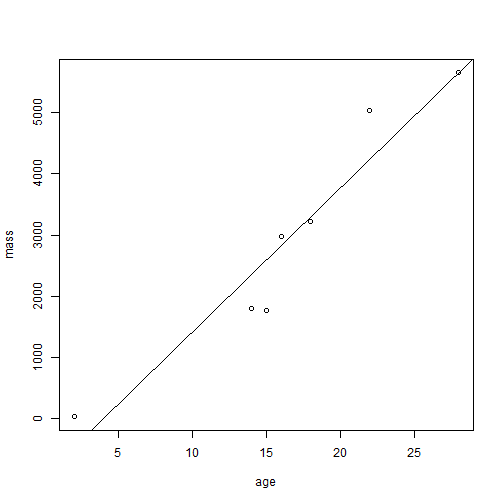## (1) Linear regression in JAGS

 #install.packages("rjags")
library(rjags)

## Loading required package: coda

## Linked to JAGS 4.2.0

## Loaded modules: basemod,bugs

 set.seed(0820)

tick <- proc.time()

model_string <- textConnection("model{
for(i in 1:n){
mass[i] ~ dnorm(beta1 + beta2*age[i],tau)
}
tau   ~  dgamma(0.01, 0.01)
beta1 ~  dnorm(0, 0.0000001)
beta2 ~  dnorm(0, 0.0000001)
}")

data  <- list(mass=mass,age=age,n=n)
inits <- list(beta1=rnorm(1),beta2=rnorm(1),tau=10)
model <- jags.model(model_string,
data = data, inits=inits, n.chains=2,
quiet=TRUE)

update(model, 10000, progress.bar="none")
samples <- coda.samples(model,
variable.names=c("beta1","beta2"),
n.iter=20000, progress.bar="none")
tock <- proc.time()
summary(samples)

##
## Iterations = 10001:30000
## Thinning interval = 1
## Number of chains = 2
## Sample size per chain = 20000
##
## 1. Empirical mean and standard deviation for each variable,
##    plus standard error of the mean:
##
##         Mean     SD Naive SE Time-series SE
## beta1 -881.2 672.87   3.3644         10.589
## beta2  232.4  37.64   0.1882          0.588
##
## 2. Quantiles for each variable:
##
##          2.5%     25%  50%    75% 97.5%
## beta1 -2197.1 -1282.8 -894 -488.6 511.2
## beta2   154.6   210.7  233  254.9 306.0

 plot(samples)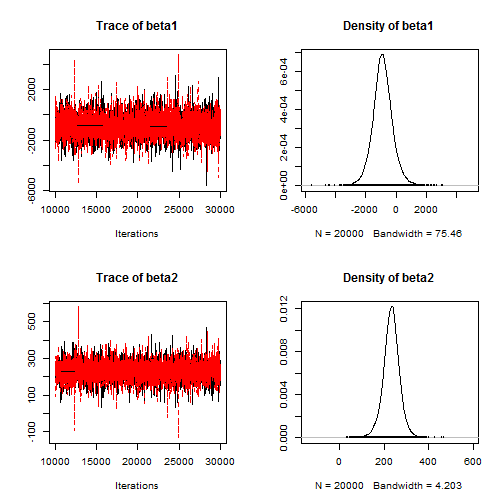effectiveSize(samples)

##    beta1    beta2
## 4038.384 4098.400

 tock-tick

## elapsed
##    0.09


## (2) Linear regression in OpenBUGS

 #install.packages("R2OpenBUGS")
library(R2OpenBUGS)

## Warning: package 'R2OpenBUGS' was built under R version 3.3.3

 set.seed(0820)

tick <- proc.time()
model_string <- function(){
for(i in 1:n){
mass[i] ~ dnorm(mn[i],tau)
mn[i]  <- beta1 + beta2*age[i]
}
tau   ~  dgamma(0.01, 0.01)
beta1 ~  dnorm(0, 0.0000001)
beta2 ~  dnorm(0, 0.0000001)
}

data  <- list(mass=mass,age=age,n=n)
inits <- function(){list(beta1=rnorm(1),beta2=rnorm(1),tau=10)}
fit   <- bugs(model.file=model_string,
data=data,inits=inits,
parameters.to.save=c("beta1","beta2"),
n.chains=2,n.iter=30000,n.burnin=10000)
tock <- proc.time()
fit

## Inference for Bugs model at "C:/Users/BJREIC~1.WOL/AppData/Local/Temp/Rtmpm8JQjK/model13201b13212e.txt",
## Current: 2 chains, each with 30000 iterations (first 10000 discarded)
## Cumulative: n.sims = 40000 iterations saved
##            mean    sd    2.5%     25%    50%    75% 97.5% Rhat n.eff
## beta1    -883.1 701.1 -2255.0 -1296.0 -893.4 -480.7 562.5    1  1600
## beta2     232.4  39.2   152.2   209.6  233.0  255.5 309.1    1  1600
## deviance  111.3   3.1   107.8   109.0  110.5  112.6 119.3    1 11000
##
## For each parameter, n.eff is a crude measure of effective sample size,
## and Rhat is the potential scale reduction factor (at convergence, Rhat=1).
##
## DIC info (using the rule, pD = Dbar-Dhat)
## pD = 3.4 and DIC = 114.7
## DIC is an estimate of expected predictive error (lower deviance is better).

 plot(fit)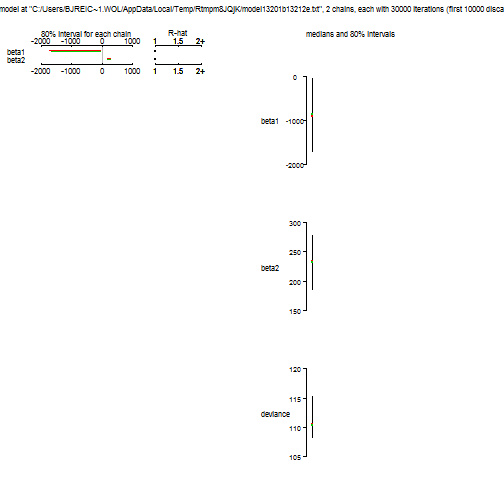tock-tick

## elapsed
##    3.75


## (3) Linear regression in STAN

 #install.packages("rstan")
library(rstan)

## Warning: package 'rstan' was built under R version 3.3.3

## Loading required package: ggplot2

## Loading required package: StanHeaders

## Warning: package 'StanHeaders' was built under R version 3.3.3

## rstan (Version 2.17.3, GitRev: 2e1f913d3ca3)

## For execution on a local, multicore CPU with excess RAM we recommend calling
## options(mc.cores = parallel::detectCores()).
## To avoid recompilation of unchanged Stan programs, we recommend calling
## rstan_options(auto_write = TRUE)

##
## Attaching package: 'rstan'

## The following object is masked from 'package:R2OpenBUGS':
##
##     monitor

## The following object is masked from 'package:coda':
##
##     traceplot

 set.seed(0820)

tick <- proc.time()
stan_model <- "

data {
int<lower=0> n;
vector [n] mass;
vector [n] age;
}

parameters {
real beta1;
real beta2;
real<lower=0> sigma;
}

model {
vector [n] mu;
beta1 ~ normal(0,1000000);
beta2 ~ normal(0,1000000);
sigma ~ cauchy(0.0,1000);
mu    = beta1 + beta2*age;
mass  ~ normal(mu,sigma);
}
"

data <- list(n=n,age=age,mass=mass)
fit_stan <- stan(model_code = stan_model,
data = data,
chains=2, warmup = 10000, iter = 30000)

## In file included from C:/Program Files/R/R-3.3.2/library/BH/include/boost/config.hpp:39:0,
##                  from C:/Program Files/R/R-3.3.2/library/BH/include/boost/math/tools/config.hpp:13,
##                  from C:/Program Files/R/R-3.3.2/library/StanHeaders/include/stan/math/rev/core/var.hpp:7,
##                  from C:/Program Files/R/R-3.3.2/library/StanHeaders/include/stan/math/rev/core/gevv_vvv_vari.hpp:5,
##                  from C:/Program Files/R/R-3.3.2/library/StanHeaders/include/stan/math/rev/core.hpp:12,
##                  from C:/Program Files/R/R-3.3.2/library/StanHeaders/include/stan/math/rev/mat.hpp:4,
##                  from C:/Program Files/R/R-3.3.2/library/StanHeaders/include/stan/math.hpp:4,
##                  from C:/Program Files/R/R-3.3.2/library/StanHeaders/include/src/stan/model/model_header.hpp:4,
##                  from file132029834325.cpp:8:
## C:/Program Files/R/R-3.3.2/library/BH/include/boost/config/compiler/gcc.hpp:186:0: warning: "BOOST_NO_CXX11_RVALUE_REFERENCES" redefined
##  #  define BOOST_NO_CXX11_RVALUE_REFERENCES
##  ^
## <command-line>:0:0: note: this is the location of the previous definition
## In file included from C:/Program Files/R/R-3.3.2/library/StanHeaders/include/stan/math/rev/core.hpp:44:0,
##                  from C:/Program Files/R/R-3.3.2/library/StanHeaders/include/stan/math/rev/mat.hpp:4,
##                  from C:/Program Files/R/R-3.3.2/library/StanHeaders/include/stan/math.hpp:4,
##                  from C:/Program Files/R/R-3.3.2/library/StanHeaders/include/src/stan/model/model_header.hpp:4,
##                  from file132029834325.cpp:8:
## C:/Program Files/R/R-3.3.2/library/StanHeaders/include/stan/math/rev/core/set_zero_all_adjoints.hpp:14:17: warning: 'void stan::math::set_zero_all_adjoints()' defined but not used [-Wunused-function]
##      static void set_zero_all_adjoints() {
##                  ^
##
## SAMPLING FOR MODEL 'a21dfc9ecf8d94d8bb63d64a7fe0d210' NOW (CHAIN 1).
##
## Gradient evaluation took 0 seconds
## 1000 transitions using 10 leapfrog steps per transition would take 0 seconds.
## Adjust your expectations accordingly!
##
##
## Iteration:     1 / 30000 [  0%]  (Warmup)
## Iteration:  3000 / 30000 [ 10%]  (Warmup)
## Iteration:  6000 / 30000 [ 20%]  (Warmup)
## Iteration:  9000 / 30000 [ 30%]  (Warmup)
## Iteration: 10001 / 30000 [ 33%]  (Sampling)
## Iteration: 13000 / 30000 [ 43%]  (Sampling)
## Iteration: 16000 / 30000 [ 53%]  (Sampling)
## Iteration: 19000 / 30000 [ 63%]  (Sampling)
## Iteration: 22000 / 30000 [ 73%]  (Sampling)
## Iteration: 25000 / 30000 [ 83%]  (Sampling)
## Iteration: 28000 / 30000 [ 93%]  (Sampling)
## Iteration: 30000 / 30000 [100%]  (Sampling)
##
##  Elapsed Time: 0.325 seconds (Warm-up)
##                0.594 seconds (Sampling)
##                0.919 seconds (Total)
##
##
## SAMPLING FOR MODEL 'a21dfc9ecf8d94d8bb63d64a7fe0d210' NOW (CHAIN 2).
##
## Gradient evaluation took 0 seconds
## 1000 transitions using 10 leapfrog steps per transition would take 0 seconds.
## Adjust your expectations accordingly!
##
##
## Iteration:     1 / 30000 [  0%]  (Warmup)
## Iteration:  3000 / 30000 [ 10%]  (Warmup)
## Iteration:  6000 / 30000 [ 20%]  (Warmup)
## Iteration:  9000 / 30000 [ 30%]  (Warmup)
## Iteration: 10001 / 30000 [ 33%]  (Sampling)
## Iteration: 13000 / 30000 [ 43%]  (Sampling)
## Iteration: 16000 / 30000 [ 53%]  (Sampling)
## Iteration: 19000 / 30000 [ 63%]  (Sampling)
## Iteration: 22000 / 30000 [ 73%]  (Sampling)
## Iteration: 25000 / 30000 [ 83%]  (Sampling)
## Iteration: 28000 / 30000 [ 93%]  (Sampling)
## Iteration: 30000 / 30000 [100%]  (Sampling)
##
##  Elapsed Time: 0.317 seconds (Warm-up)
##                0.678 seconds (Sampling)
##                0.995 seconds (Total)

 tock <- proc.time()
tock-tick

## elapsed
##      32

 fit_stan

## Inference for Stan model: a21dfc9ecf8d94d8bb63d64a7fe0d210.
## 2 chains, each with iter=30000; warmup=10000; thin=1;
## post-warmup draws per chain=20000, total post-warmup draws=40000.
##
##          mean se_mean     sd     2.5%      25%     50%     75%   97.5%
## beta1 -920.31    7.22 735.77 -2392.15 -1339.97 -918.21 -500.08  552.45
## beta2  234.46    0.40  40.84   153.34   211.22  234.34  258.03  315.91
## sigma  746.45    2.86 288.17   401.71   555.14  682.05  860.56 1466.96
## lp__   -43.13    0.02   1.52   -47.04   -43.80  -42.73  -42.03  -41.41
##       n_eff Rhat
## beta1 10391    1
## beta2 10360    1
## sigma 10187    1
## lp__   8400    1
##
## Samples were drawn using NUTS(diag_e) at Thu Sep 13 08:47:35 2018.
## For each parameter, n_eff is a crude measure of effective sample size,
## and Rhat is the potential scale reduction factor on split chains (at
## convergence, Rhat=1).

 plot(fit_stan)

## ci_level: 0.8 (80% intervals)

## outer_level: 0.95 (95% intervals)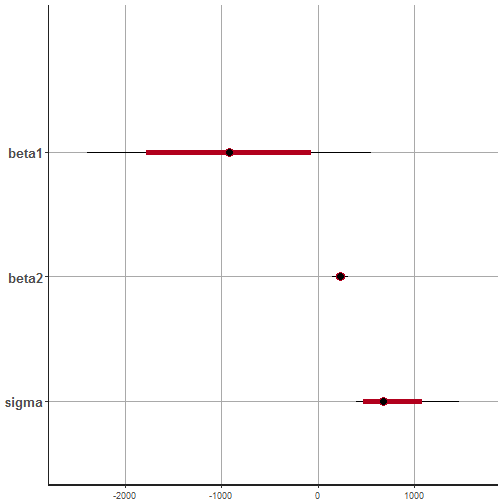## (4) Linear regression in NIMBLE

 library(nimble)

## nimble version 0.6-11 is loaded.
## For more information on NIMBLE and a User Manual,
## please visit http://R-nimble.org.

##
## Attaching package: 'nimble'

## The following object is masked from 'package:stats':
##
##     simulate

 set.seed(0820)

model_string <- nimbleCode({
for(i in 1:n){
mass[i] ~ dnorm(mn[i],tau)
mn[i]  <- beta1 + beta2*age[i]
}
tau   ~  dgamma(0.01, 0.01)
beta1 ~  dnorm(0, 0.0000001)
beta2 ~  dnorm(0, 0.0000001)
})

consts   <- list(n=n,age=age)
data     <- list(mass=mass)
inits    <- function(){list(beta1=rnorm(1),beta2=rnorm(1),tau=10)}
samples  <- nimbleMCMC(model_string, data = data, inits = inits,
constants=consts,
monitors = c("beta1", "beta2"),
samplesAsCodaMCMC=TRUE,WAIC=FALSE,
niter = 30000, nburnin = 10000, nchains = 2)

## defining model...

## building model...

## setting data and initial values...

## running calculate on model (any error reports that follow may simply
## reflect missing values in model variables) ...

##

## checking model sizes and dimensions...

##

## checking model calculations...

## model building finished.

## compiling... this may take a minute. Use 'showCompilerOutput = TRUE' to see C++ compiler details.

## compilation finished.

## running chain 1...

## |-------------|-------------|-------------|-------------|
## |-------------------------------------------------------|

## running chain 2...

## |-------------|-------------|-------------|-------------|
## |-------------------------------------------------------|

 tock <- proc.time()
tock-tick

## elapsed
##   48.02

 plot(samples)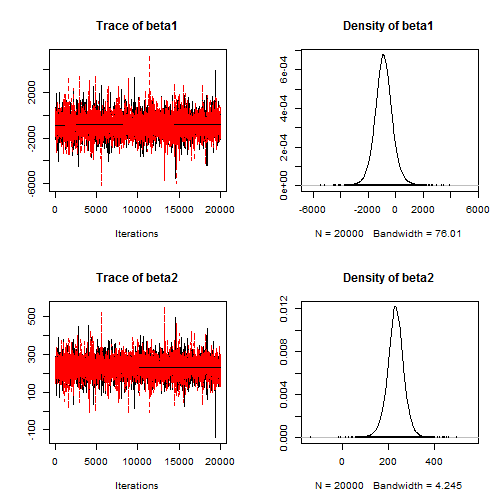effectiveSize(samples)

##    beta1    beta2
## 3983.387 4005.606